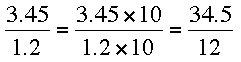SEARCH HOMEMath Central Quandaries & QueriesQuestion from Brooklyn, a student: I am studying for an exam, and I will not be able to use a calculator. I can not figure out how to do division is the number on the outside of the box is a decimal. Ex: 2/6.3=X Can you please help me figure this out?Hi Brooklyn,

The key is to write an equivalent fraction with an integer in the denominator. Thus if you want to find the decimal representation of 3.45/1.2 you writeNow long division will give 3.45/1.2 = 2.875.

PennyMath Central is supported by the University of Regina and The Pacific Institute for the Mathematical Sciences.